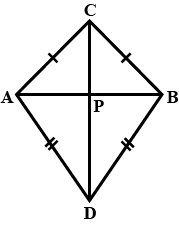State whether the given statement is true or false.AB is a line segment. C and D are points on opposite sides of AB such that each of them is equidistant from the point A and B as in the figure. The line CD is the perpendicular bisector of AB.

# State whether the given statement is true or false.AB is a line segment. C and D are points on opposite sides of AB such that each of them is equidistant from the point A and B as in the figure. The line CD is the perpendicular bisector of AB.1. A
True
2. B
False

Fill Out the Form for Expert Academic Guidance!l

+91

Live ClassesBooksTest SeriesSelf Learning

Verify OTP Code (required)

### Solution:

The given statement is true.
Concept- We solve this problem by applying the ⁷concept of congruent triangles to obtain the necessary condition, i.e., to prove the perpendicular bisector.
Here,is equidistant from pointsand.andis equidistant from pointsandWe have to prove thatandInand(1)common)..... (SSS congruence rule)
Hence,....... (C.P.C.T) ….(3)
In(SAS congruence rule)C.P.C.T)(C.P.C.T)
Asis a line segment,Hence,andisbisector of.
​Hence, the given statement is true.

## Related content

 Area of Square Area of Isosceles Triangle Pythagoras Theorem Triangle Formula Perimeter of Triangle Formula Area Formulae Volume of Cone Formula Matrices and Determinants_mathematics Critical Points Solved Examples Type of relations_mathematics+91

Live ClassesBooksTest SeriesSelf Learning

Verify OTP Code (required)